Image Files -  click on the thumbnail to see larger image N = 3 N = 4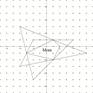N = 5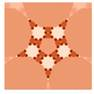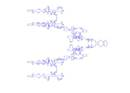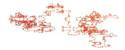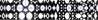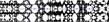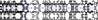N = 6 N = 7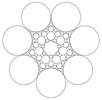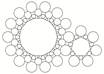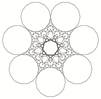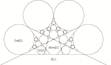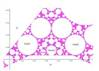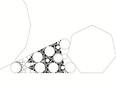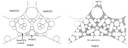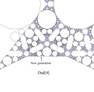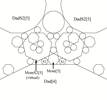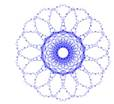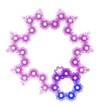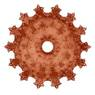N = 8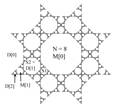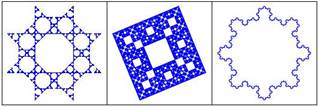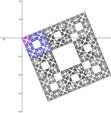N = 9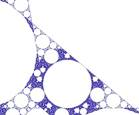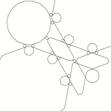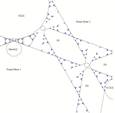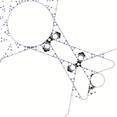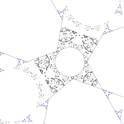N = 10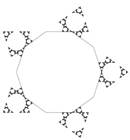N = 11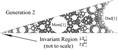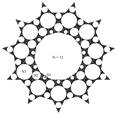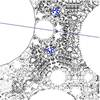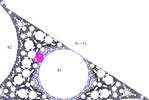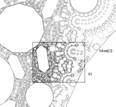N = 12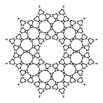N = 13 N = 14 woven N = 16 N = 17 N = 281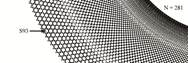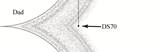Julia Durer Misc.